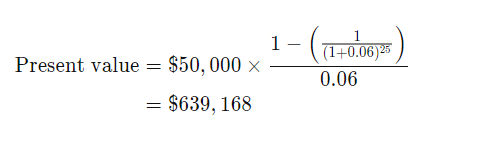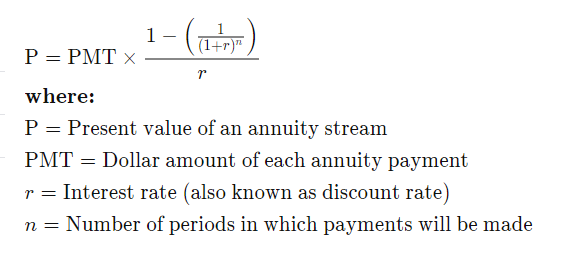# Present value of annuity calculation

Hi I am trying to calculate present value of anuuity using math formulae, but I am unable to put below formulae in right syntax, can any one help here or suggest any alternative for calculating Present value. Knime doesnt seem to have any Finance related functions.

PV of annuity :Original formula :I have tried to put the values but getting incorrect results :

Interest rate : 0.015 per period
Period : 12 months

This worked for me using your initial example:

`\$PMT\$ * (1-(1/((1+\$r\$)^\$n\$)))/\$r\$`

Where PMT, r, and n were all defined from an input table. To make it a bit more readable using your second inputs:

`\$Proposed EMI\$ * (1-(1/((1+0.015)^12)))/0.015`

2 Likes

thanks, i was struggling with the syntax

1 Like

This topic was automatically closed 7 days after the last reply. New replies are no longer allowed.